### 13.4.3.2 Nonconservative forces

The Lagrangian formulation has been extended so far to handle constraints onthat lower the dimension of the tangent space. The formulation can also be extended to allow nonconservative forces. The most common and important example in mechanical systems is friction. The details of friction models will not be covered here; see . As examples, friction can arise when bodies come into contact, as in the joints of a robot manipulator, and as bodies move through a fluid, such as air or water. The nonconservative forces can be expressed as additional generalized forces, expressed in an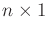vector of the form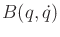. Suppose that an action vector is also permitted. The modified Euler-Lagrange equation then becomes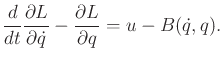(13.181)

A common extension to (13.142) is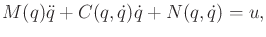(13.182)

in which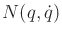generalizes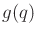to include nonconservative forces. This can be generalized even further to include Pfaffian constraints and Lagrange multipliers,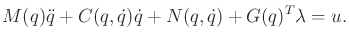(13.183)

The Lagrange multipliers become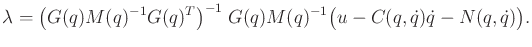(13.184)

Once again, the phase transition equation can be derived in terms of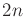phase variables and generalizes (13.148).

Steven M LaValle 2020-08-14